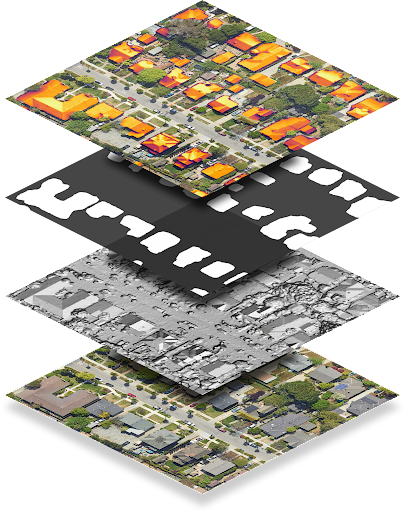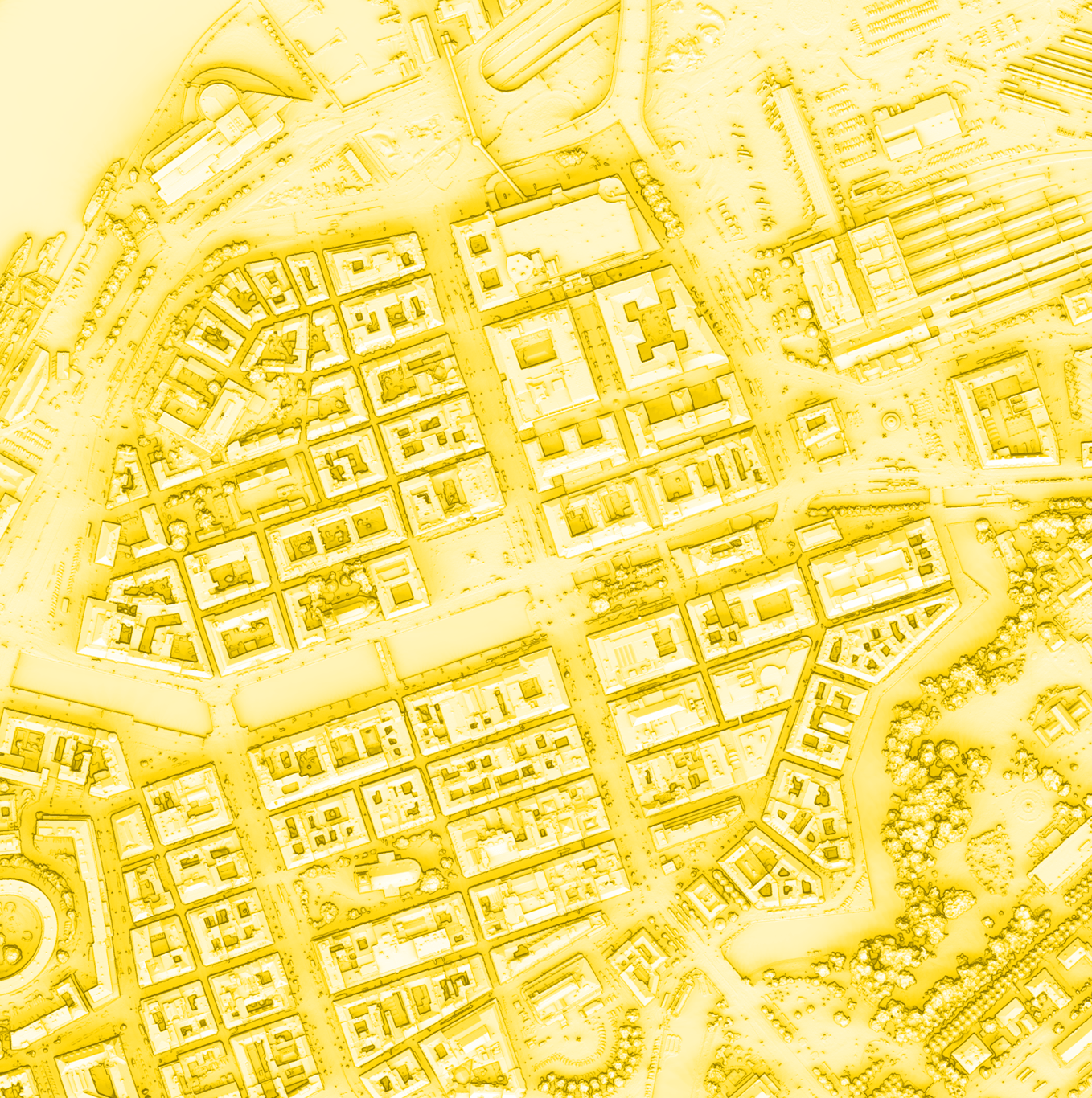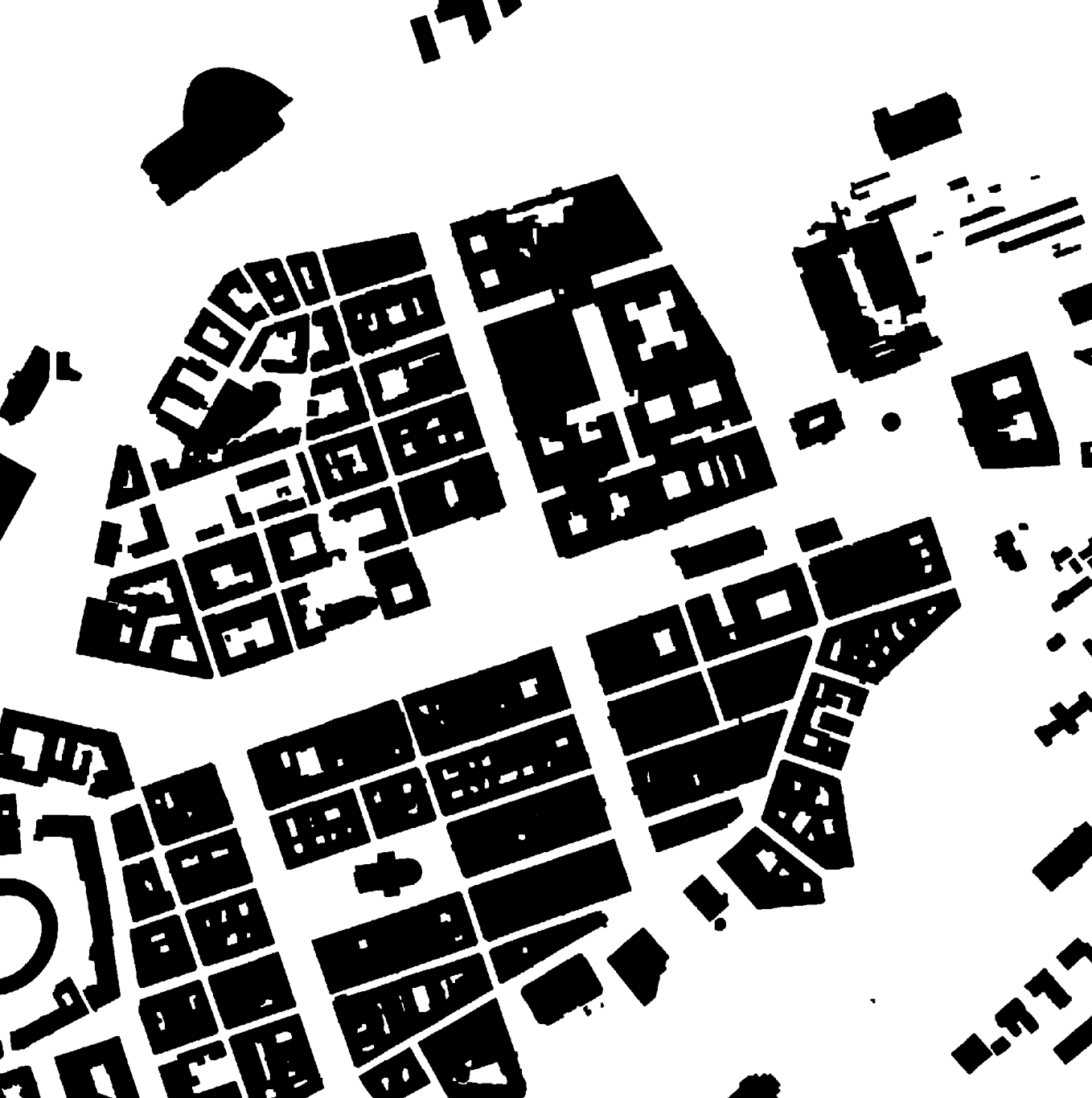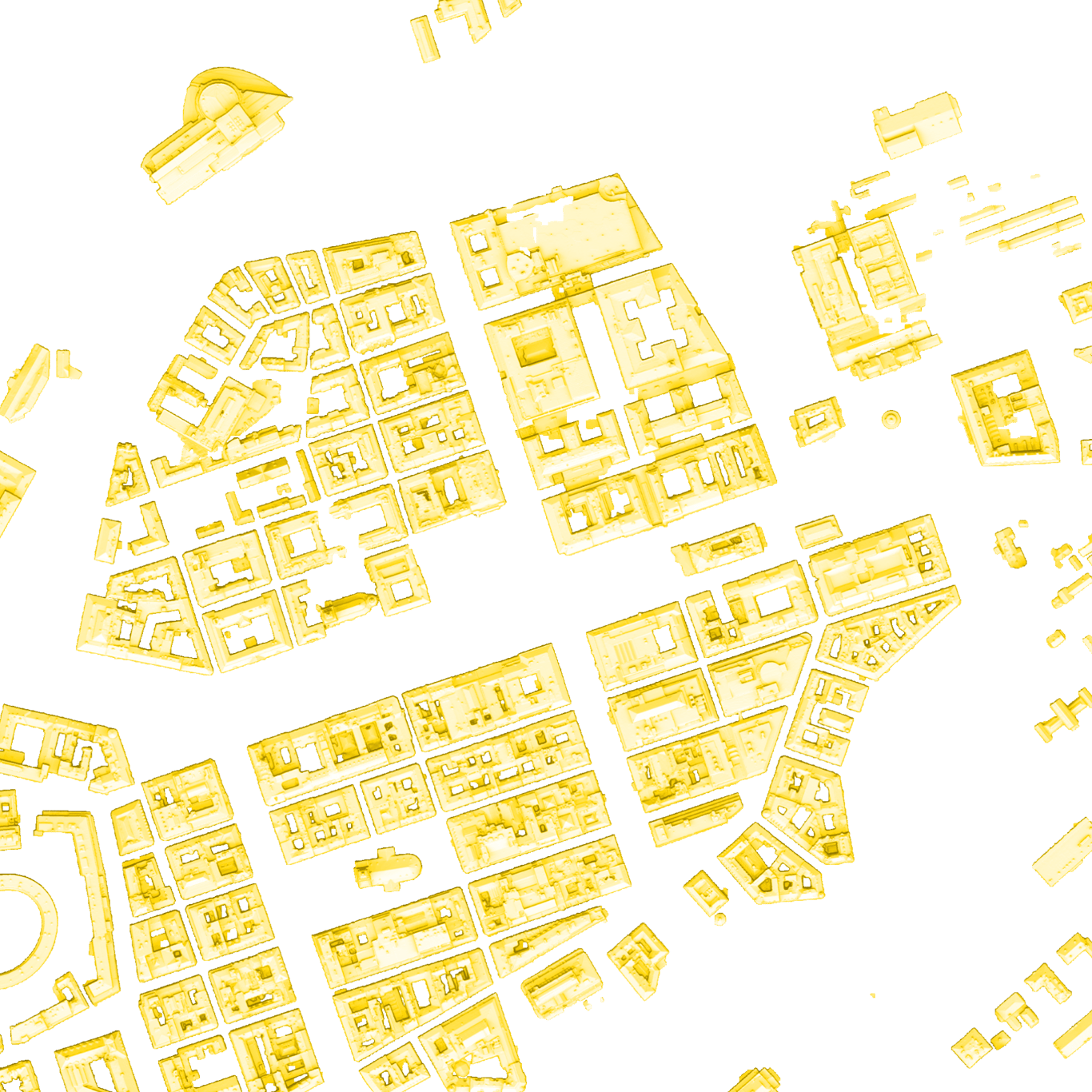3 months ago

## Integrating the new Solar API in Google Maps

The new Solar API from Google Maps Platform was just released the other day. This is how you can integrate it with dynamic maps!

Google Maps released the API with a demo as usual. In this article, I'm going to focus on the third slide where they overlay the GeoTIFF on a Google Maps instance.

The two packages I used in my experimentations is geotiff.js - for decoding the GeoTIFF data, and geolib by manuelbieh to calculate latitude and longitude center based boundary.

The geolib package can be replaced by the spherical geometry library's computeOffset function by computing to all 4 directions.

First I made an interface for calling the new Solar REST API, you can find my implementation in the @nora-soderlund/google-maps-solar-api package. I'm a firm believer in that you should never expose external APIs to your clients (opposed to SDKs), so I suggest that you implement an internal API as a proxy for your clients instead of calling the external API (Solar API) directly in the client. This is only done for experimentation purposes in the demo repository.

I'm not going to focus on all the options you can tweak for different responses, or how the date based flux works; I'm primarily focusing on implementing the Solar API as an overlay to an Google Maps instance in this article.The first layer we want to introduce is the flux layer, which is actually the last layer (first one in the top-to-bottom sense) shown in the image above. In my experimentations, I used specifically the annualFluxUrl layer. Given that we've fetched the flux layer endpoint, we can create a geotiff.js instance using the response's ArrayBuffer and read the raster from the first image.

const tiff = await GeoTIFF.fromArrayBuffer(tiffImageBuffer); const tiffImage = await tiff.getImage(); const tiffData = await tiffImage.readRasters();

The tiff data is an extremely large Float32Array that contains in this case (since I'm using annualFluxUrl), an kWh per kW per year value, or a value of -9999 if the Solar API is missing coverage; for each pixel, which is seperated by 0.1 meters.

For the sake of the maximum value being unknown in the sense that we could fetch more data at a later stage, we'll make the assumption that our target value is 1000 kWh per kW per year. This will represent the brightest color we can display. In a bigger scope, we'd want some kind of data layer management but for the sake of experimentations I went with a static maximum.

const maximumKwhPerKwPerYear = 1000;

You can, however, iterate each unit in this data layer if you're only showing one data layer at a time and choose the maximum value:

const maximumKwhPerKwPerYear = tiffData.reduce((unit: number, currentUnit: number) => (unit > currentUnit)?(unit):(currentUnit), 0);

The data is too much for Math.max(...) to handle, as it causes an overflow in arguments.

To read each unit as a row (y) and column (x) pixel, we can iterate through each row up until the height of the image, and each row iteration, iterate through each column until the width of the image, and in the inner scope, add the column index to the row multiplied by the width of the image. This is similar logic as when dealing with raw image data, except for there's only 1 index, instead of 4 indexes for RGBA.

You can also calculate the row and column by each index, but I found this approach to be more readable.

for(let row = 0; row < tiffData.height; row++) for(let column = 0; column < tiffData.width; column++) { const index = (row * tiffData.width) + column; const value = tiffData[index]; if(value === -9999) continue; }

To apply the fraction of the value and the maximum value to a color, I used HSL to control the lightness of a yellow hue. The minimum (0 kWh/kW/year) being 50deg 100% 0% and the maximum (>1000 kWh/kW/year) being 50deg 100% 100%.

context.fillStyle = `hsl(50 100% \${((value / maximumKwhPerKwPerYear) * 100)}%)`; context.fillRect(column, row, 1, 1);

This results in a canvas that looks like this:With the flux layer done, we can now continue with the second layer we're doing to do, which is the roof top mask layer. We don't have to do this, but depending on how you want the results to be, we're going to remove everything but the roof tops. This is useful in e.g. solar panel planning visualizations, and other data visualizations.

Regardless of what it's for, we're going to follow the same steps as earlier, except for we only need to check for positive values, and then draw the mask layer on top of the existing layers (the flux canvas) with a destination-in composite operation, which will only keep the pixels that are not transparent in the new image (the mask layer).

For context, I'm processing each layer on its own canvas asynchronously, then drawing them on a main canvas afterwards.

const tiffImageBuffer = await getTiff(dataLayers.maskUrl!); const tiff = await GeoTIFF.fromArrayBuffer(tiffImageBuffer); const tiffImage = await tiff.getImage(); const tiffData = await tiffImage.readRasters(); const canvas = document.createElement("canvas"); canvas.width = tiffData.width; canvas.height = tiffData.height; const context = canvas.getContext("2d") as CanvasRenderingContext2D; for(let row = 0; row < tiffData.height; row++) for(let column = 0; column < tiffData.width; column++) { const index = (row * tiffData.width) + column; if(tiffData[index]) context.fillRect(column, row, 1, 1); }

This results in a canvas that looks like this:Now we can draw these layers on our main canvas, and we should get a result like this:

context.drawImage(fluxCanvas, 0, 0, fluxCanvas.width, fluxCanvas.height, 0, 0, size, size); context.globalCompositeOperation = "destination-in"; context.drawImage(maskCanvas, 0, 0, maskCanvas.width, maskCanvas.height, 0, 0, size, size);Now for the final step of getting this final layer onto our map, we'll almost follow the custom overlay guide to the tee. First we have to calculate the bounds of our tile.

Using the getBoundsOfDistance function from the geolib package I mentioned earlier, we can pass through the same center coordinates as to the data layers endpoint, and the same radius parameter, then we can create a new LatLngBounds instance and extend it with each boundary coordinate from the getBoundsOfDistance function:

const radius = 500; const coordinateBounds = getBoundsOfDistance( coordinate, radius ); const dataLayers = await getDataLayers({ location: coordinate, radiusMeters: radius, view: layer, pixelSizeMeters: 0.5 }); const bounds = new google.maps.LatLngBounds(); coordinateBounds.forEach((coordinate) => { bounds.extend({ lat: coordinate.latitude, lng: coordinate.longitude }); });

Now we can create an OverlayView instance that follows the linked guide. Instead of passing a div, we'll pass our canvas element.

It's as simple as that!

Since there's a tiny mismatch between the static map and the dynamic satellite map, you can throw in a border-box border and draw the RGB layer behind all other layers, resulting in the map in the beginning of this article.

The next step would be integrating these overlays in a way that joins together smoothly unless you only want to capture a specific area (such as an address). Loading data layers with all this data is a heavy task and the tiff endpoints are not very quick (yet at least). This method also works for integrating the Solar API on a static map.

To use this method with a static map, you can use the rgbUrl imagery layer as the very base layer. You can draw it at the very end by using a destination-over composite operation. Instead of reading the values, you can use the image data right away on the canvas.

Visualizing potential solar panel placements in Google Maps